# Decimal calculator

## Result:

### 7 / 100 = 0.07

Spelled result in words is zero point zero seven.

### Calculation steps

1. Divide: 7 / 100 = 0.07
The calculator makes basic and advanced operations with decimals, real numbers and integers. It also shows detailed step-by-step information about calculation procedure. Solve problems with two, three or more decimals in one expression. Add, subtract and multiply decimals step-by-step. This calculator uses addition, subtraction, multiplication or division for calculations on positive or negative decimal numbers, integers, real numbers and whole numbers. This online decimals calculator will help you understand how to add, subtract, multiply or divide decimals.The calculator follows well-known rules for order of operations. The most common mnemonics for remembering this order of operations are:
PEMDAS - Parentheses, Exponents, Multiplication, Division, Addition, Subtraction.
BEDMAS - Brackets, Exponents, Division, Multiplication, Addition, Subtraction
BODMAS - Brackets, Of or Order, Division, Multiplication, Addition, Subtraction.
GEMDAS - Grouping Symbols - brackets (){}, Exponents, Multiplication, Division, Addition, Subtraction.
Be careful, always do multiplication and division before addition and subtraction. Some operators (+ and -) and (* and /) has the same priority and then must evaluate from left to right.

#### Examples:

addition of decimals: -1.5 + 2.45
multiplying decimals: 0.25 * 0.2
division of a decimals: 5.2 / 0.5
parenthesis: 3 + 7{4 + 3[2-(8 - 4)×3] + 5}×2
2nd power and order of evaluation: 6^2-(2*7+4^2)
cube root: cuberoot(27)
Convert fraction to a decimal: 3/4
decimals and mixed numbers: 1.5 - 1 1/5

## Decimals in word problems:

• Expanded formWhat is the expanded form of 0.21?
• Rolls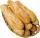Mom bought 13 rolls. Dad ate 3.5 rolls. How many rolls left when Peter yet put two at dinner?
• RatioIncrease in the ratio 20:4 number 18.5.
• Ratio v2Decrease in the ratio 12:16 number 13.2.
• Lowest termsReduce to the lowest terms: 32/124
• Package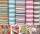The package was 23 meters of textile. The first day sold 12.3 meters. How many meters of textile remained in the package?
• Tenths digit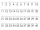For 10.932, which digit is in the tenths place?
• Whole numbersPavol wrote down a number that is both rational and a whole number. What is one possible number she could have written down?
• JagdeepJagdeep had a strip of paper measuring 1.8 m. He cut it into 4 equal strips. How long is each shorter strip of paper?
• Bureaucracy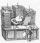23 people attended the office. 9 of them completed the questionnaire. What is the percentage of all people?
• Zhiwei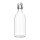Zhiwei wants to divide 19 liters of water equally into 6 pots. Find the volume of water in each pot?
• Find the 21Find the sum of the six terms of the finite geometric sequence 96, -48, 24, -12
• Distributive propertyVerify the distributive property a×(b+c)=(a×b)+(a*c) for the rational number a=5/8, b=7/4 and c=2/3
• Double 5Peter was thinking of a number. Peter doubles it and gets an answer of 8.6. What was the original number?
• On the tripOn the trip were more than 55 children, but less than 65 children. Groups of 7 could be formed, but groups of 8 no. How many children were on a trip?
• What is one third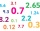What is 1/3 as a decimal? Give your answer rounded to 2 decimal places.
• Thousandths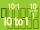If you have 0.08 what is the form in thousandths?